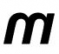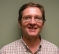Started by 7 years ago9 replieslatest reply 7 years ago1967 views

Hello All,

I would like to get some clarification wrt to the #LTE downlink process at the Basestation side.

I am using a 20 MHz system to be specific in my questions.

It is mentioned that in a 20 MHz system, there are 1200 sub-carriers, The OFDM is typically implemented using a 2048 pt. iFFT. Each point in the iFFT corresponds to 1 sub-carrier. It is mentioned that out of 2048 points, 848 sub-carriers are padded with 0.

So, where does 0 padding happen? Is this at the PHY or at the upper layers?

Does 0 padding happen for specific sub-carriers or it does not matter?

As I understand, the iFFT process is meant to convert frequency domain to time domain. I far I understand, the data data coming to iFFT stage is just Layer 2 data. So, I am unable to understand where the data is converted into frequency domain. Need comment please.

Conceptually, I don't understand sample rate. Initially, I confused sample and sampling rate (as in Nyquist rate) but still I don't get how sample rate comes into picture.

Also, using 15 KHz subcarrier frequency and 2048 sub-carriers, the sample rate of a 20 MHz system is given as 30.72 MHz. So, I am further confused as to what a sample rate of 30.72 MHz in a 20 MHz system means.

One more question, where is 20 MHz in a 20 MHz system coming into picture? Is it based on number of sub-carriers that is decided? A related question is, what parameters in an LTE system can be conceptually seen as independent parameters and what are derived from them? For example, is number of sub-carriers the parameter from which bandwidth etc. is derived?

[ - ]In an OFDM system, data is modulated using a set of orthogonal frequencies. These orthogonal frequencies are referred to as subcarriers or FFT(or IFFT) bins.

We take the data bits(11001010011...) from the higher layer(MAC) and map them on one of modulation mapping schemes(BPSK, QPSK, QAM16, QAM64 and so on). For example, for a BPSK case we take one bit at a time and assign it a value of +1 or -1(corresponding to 1 and 0) with a normalization factor(1/sqrt(2) in this case). We do a similar kind of mapping for other modulation mapping schemes as well where instead of +/-1 we assign a value of the form I+jQ where I,Q values differ for each modulation scheme. These are also known as constellation points

These mapped symbols(or constellation points) are then arranged on the IFFT bins for one OFDM symbol and transformed from frequency domain to domain. What this means is that you are varying the amplitude and phase of the subcarrier( which is nothing but a sinusoidal wave of a particular frequency)by scaling it with a complex number. For your case we take 1200 mapped symbols and load them on some of the 2048 IFFT bins(these are generally the center 1200 points) leaving 848 IFFT bins without any data(i.e. multiplying these bins with 0). This is known as zero padding where we don't load data on some of the subcarriers, rather load 0s. This zero padding is done towards both positive and negative frequency bands(424 on each positive and negative end of the 2048 point IFFT) to ensure that data from an OFDM doesn't leak into the subsequent OFDM symbol. So, yes zero padding is done in the Physical Layer.

Regards,

Prashant Sharma

[ - ]Hello Prashant,

This zero padding is done towards both positive and negative frequency bands(424 on each positive and negative end of the 2048 point IFFT) to ensure that data from an OFDM doesn't leak into the subsequent OFDM symbol.

I assume the extreme ends of the subcarriers are padded with 0. Otherwise, the signal bandwidth would still be 20 MHz with certain frequency bands empty.

Is this correct understanding?

Thanks.

[ - ]The 20MHz LTE must be sampled physically at 30.72MHz then it results in 18.0MHz bandwidth (nominally known as 20MHz LTE), you can work out bandwidth as follows:

30.72*1200/2048 = 18.0MHz

only 1200 fft points are active, the rest are dead and the active points are chosen around zero bin symmetrically.

Your post is not clear when you talk about sample/sample rate then suddenly 15MHz comes into picture.

Kaz

[ - ](OFDM symbol period) = 1 / (subcarrier spacing * IFFT size) or

Sampling clock frequency = subcarrier spacing * IFFT size

15kHz * 2048 = 30.72 MHz.

As pointed above, portion of carriers is used for transmission with other set to zero. That reduces useful bandwidth comparing to full bandwidth of 30.72 MHz. Reducing number of used carriers one may get narrower bandwidth with same IFFT.

[ - ]Hello rrlagic,

(OFDM symbol period) = 1 / (subcarrier spacing * IFFT size)

Thanks. OFDM symbol period is constant across system bandwidth.

Did you mean, sampling time period when you say OFDM symbol period above?

[ - ]I gave that simple formula to illustrate where 15kHz subcarrier spacing goes to.

Sure, I meant clock period. As duration of the OFDM symbol itself is fixed, one may conclude, that with narrower IFFT one may sample slower. Say, in 10 MHz system one may use 1024 IFFT with sampling frequency of 15.36 MHz. Simply speaking, the difference against 2048 case would be different number of zeroed carriers.

[ - ]Hello,

As mentioned by others, the inputs to an OFDM IFFT are in the frequency domain.  Each frequency bin (subcarrier) is populated by a complex number representing a constellation point at that frequency.  Some of the bins may be null and will be stuffed with zeros.

At the higher frequencies (the "ends") the bins have to be null so that some of the spectrum is unused (band limited).  These zeroed frequencies are needed so that the spectrum can be reproduce at a higher sample rate (up-converted).  Also, the symbol rate is unlikely to to locked to the system clock on the user equipment (UE) so these samples also need to re-sampled to the DAC clock domain.  This is done using some variation of a Farrow filter that is driven by a PLL that is precisely tracking the symbol time.  This filter also requires a band limited signal; usually less than 1/2 of the Nyquist zone.

Hope some of this makes sense.

Cheers,

Mark Napier

[ - ]Hello Napier,

Thanks. So, one question.

Before the OFDM stage, the inputs are first mapped onto constellation points and then I/Q data go into OFDM stage. So, when you say input to OFDM are in frequency domain, you mean this is happening at the QAM stage?

Also, identical data to QAM input will give identical output. As we always say that input to OFDM is in frequency domain, the way I was visualizing is that data for each bin, at the input of OFDM, when seen in frequency domain, would occupy frequency band pertaining to the corresponding sub-carrier frequency.

Where Am I going wrong conceptually?

[ - ]